# Modeling metal–semiconductor contacts: The Ag–Si interface¶

Version: 2016.1

Contacts between metals and doped semiconductors are ubiquitous in modern technology and find applications in several important areas, e.g. photovoltaics. QuantumATK provides an excellent toolbox to study such interfaces, because it correctly accounts for the semiconductor band gap and doping, and describes the interface using the physically correct boundary conditions.

This case study shows how the properties of metal–semiconductor interfaces can be calculated and analyzed using QuantumATK and the NEGF (non-equilibrium Green’s function) method. To this end, we will reproduce the main calculations and theoretical analyses reported in the recent publication “General atomistic approach for modeling metal–semiconductor interfaces using density functional theory and non-equilibrium Green’s function” [cSMB+16], which considers the Ag–Si interface. Notice, however, that the methods presented here are completely general and can be applied to any metal–semiconductor interface.

You will be guided through the following steps:

Note

QuantumWise case studies are primarily directed at experienced users of QuantumATK. Instructions are deliberately concise in order to focus mostly on the science.

For more basic details on how to use QuantumATK, please refer to the tutorials Introduction to QuantumATK and Transport calculations with QuantumATK.

Warning

If you are running this case study with QuantumATK v2017.2 or higher, be aware that you will have to set explicitly the electronic temperature to $$T = 300\ \mathrm{K}$$ in all the electronic structure calculations performed for either a BulkConfiguration or for a DeviceConfiguration, in order to reproduce the results presented in the case study.## Creating the device¶

Tip

If you wish to skip this part, you can just download the device configuration as an QuantumATK Python script: device.py.

The first step is to relax the bulk structures of silver and silicon. Build the structures using the database in theBuilder and send them both to the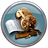Scripter, one at a time. In both scripts, add aNew Calculator block with the following settings:

• ATK-DFT
• LDA exchange-correlation functional
• 11x11x11 k-point sampling

Also add the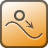OptimizeGeometry block, set the force and stress tolerances to 0.01 eV/Å and 0.001 eV/Å3, respectively, and uncheck the Constrain cell box. Run both scripts to obtain the optimized structures. The calculations can easily be run on your local machine.

The Ag and Si (100) surfaces can now by created using the Surface (Cleave) tool in the Builder, and from these you can create the interface as explained in the tutorial Building an interface between Ag(100) and Au(111). You should include 6 layers of Ag and 9 layers of Si. Choose Strain first surface in the Select Surface Cells window under Straining method and choose the cell shown in the figure below.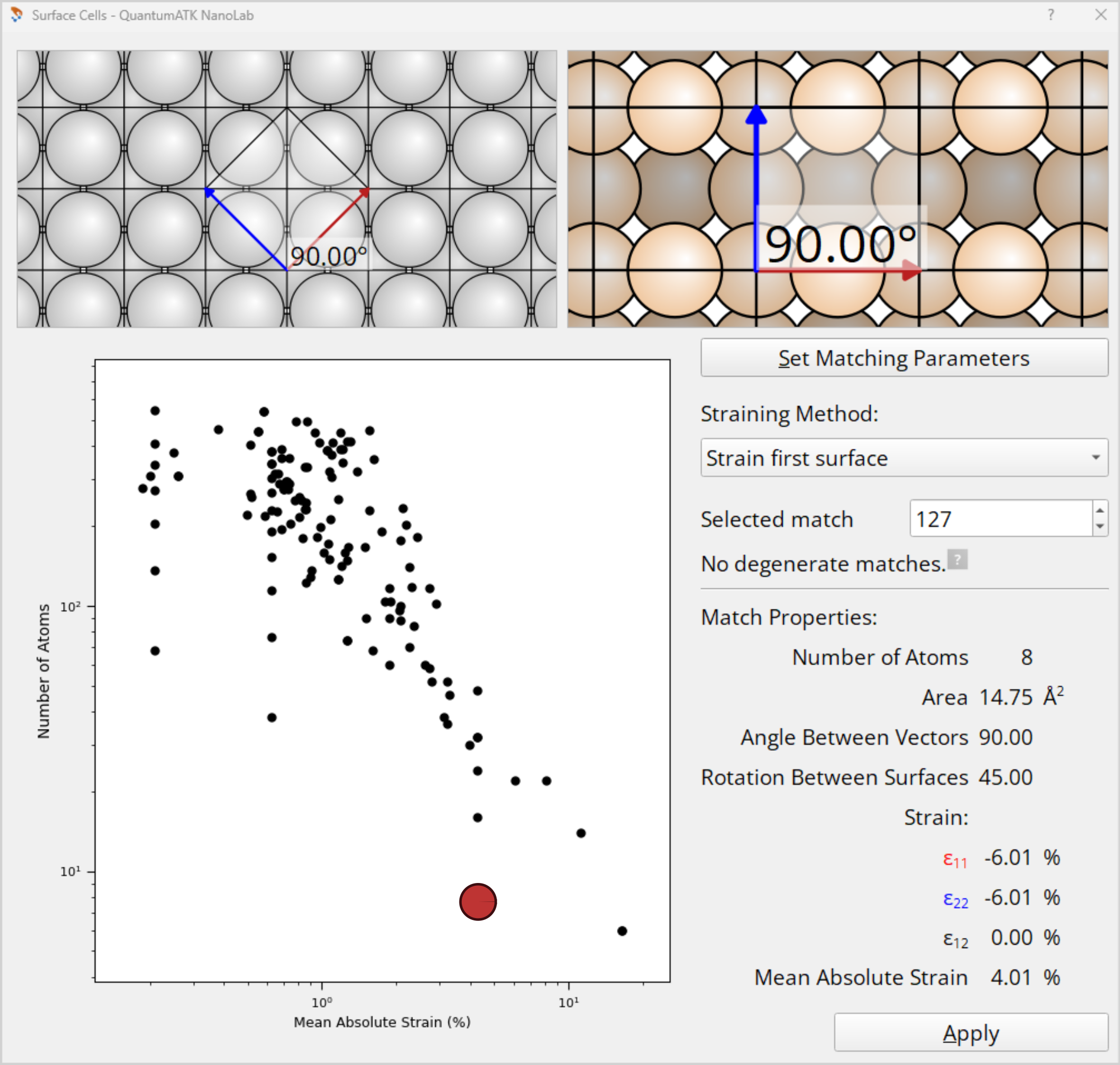Tip

You can learn more about the Interface Builder in the Technical Notes on The Interface Builder in QuantumATK.

### Initial guesses for the interface structure¶

Use the Repeat tool to repeat the interface 2 times along the B-direction. Next, add some vacuum around the configuration by first using the Stretch Cell tool to stretch the C-vector by 30%, and then use the Center tool to center the atoms along the C-direction only.

At the Ag–Si interface, the Si dangling bonds are initially placed near the hollow site of the Ag(100) surface. In order to find the most stable interface structure, you should investigate the on top and bridge sites as well.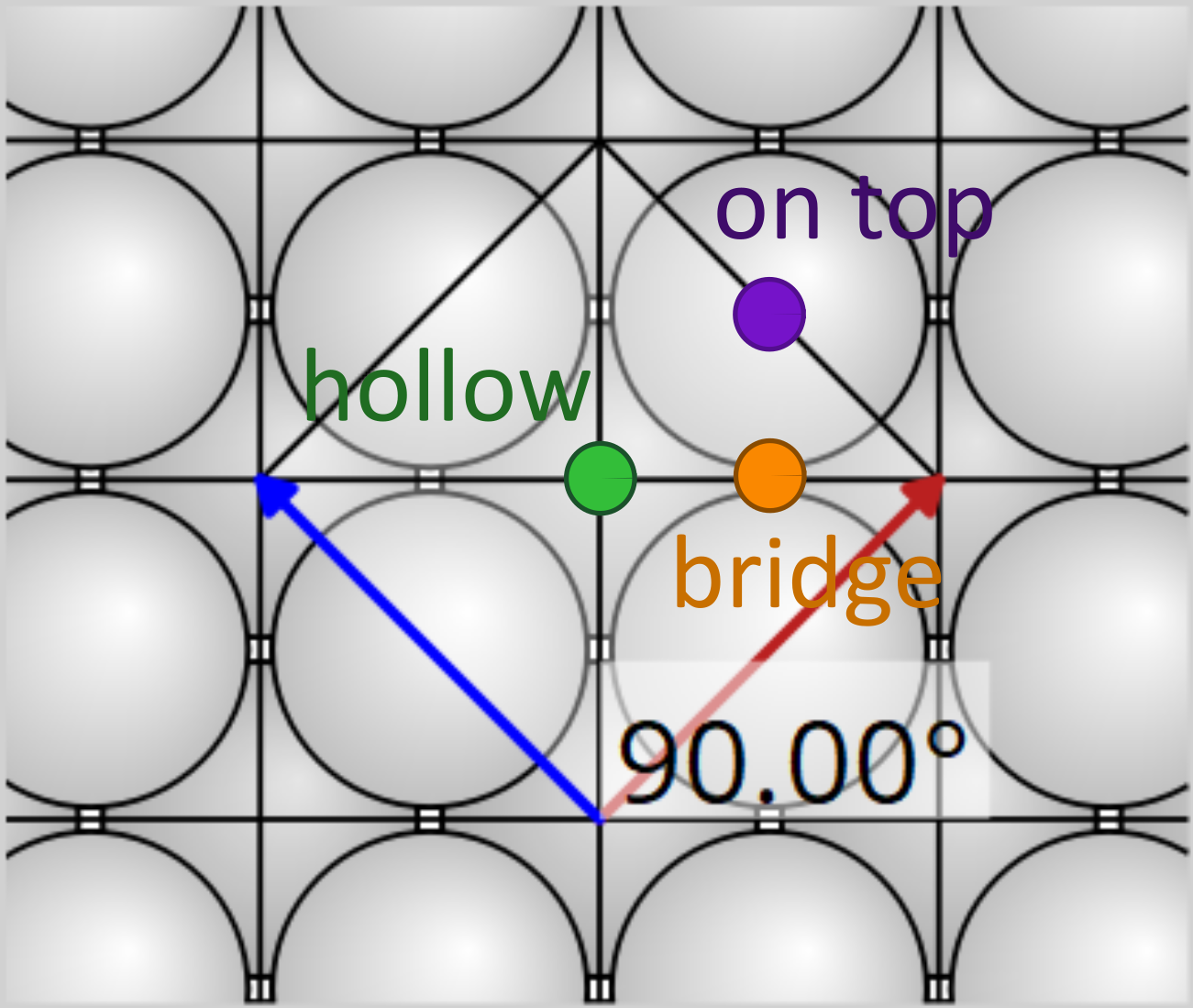Select all the Si atoms and open the Move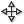tool. Choose the topmost of the Si atoms farthest to the left as an anchor by double-clicking it. Then shift the Si(100) surface 4 Å away from the Ag(100) by increasing the c-value in the Move window, and rename the structure interface_hollow. Make a copy of the structure and use the Movetool again. This time, choose the Fractional option and enter a=0.5 and b=0.75 (see image below). Name this structure interface_ontop. Finally, make a new copy, set a=0.25 and b=0.625, and name the structure interface_bridge.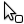Note

Repeating the structure is not necessary in the general case. We do it here in order to obtain the structural model considered in [cSMB+16].

### Electrode relaxation¶

Next, you should relax the Ag electrode in the z-direction. The electrode was strained in the x and y directions when building the interface and should therefore be allowed to relax in the z direction according to the Poisson ratio of the material. You can obtain the electrode by first creating a device configuration (apply the Device From Bulk plugin to one of the interfaces created above), and then split it using theicon. Use an ATK-DFT calculator with LDA and a 6x3x1 k-point grid, and use the same force and stress tolerance as for the bulk relaxations above. You will find that the length of the electrode C-vector increases by 9.57%. To be consistent, you should apply the same elongation to the Ag part of the three interface configurations. Use again the Stretch Cell tool: Select all the Ag atoms, enter 1.0957 for the stretch along C, and choose Selected atoms are stretched.### Central region relaxation¶

General procedures for relaxing a device configuration is explained in the tutorial Advanced device relaxation - manual workflow. Here, you will first relax the three interface structures as bulk configurations (i.e. relax the device central regions) in order to determine which of them is the most stable.

First, add some extra vacuum to all three interface structures by using the Lattice Parameters plugin to increase the C-vector length by 30%. Then use the Center tool to center the configurations along C only. Finally, use the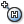Hydrogen passivation tool to passivate the dangling bonds on the Si(100) surface farthest from the interface. Note that this may add H atoms to the left of the silicon part as well as to the right. Any hydrogen placed in the interface should obviously be removed.

You are now ready to relax each configuration. Use the same calculator settings as for the Ag electrode relaxation, and use the following parameters in theOptimizeGeometry block:

• 0.02 eV/Å force tolerance.
• Do not enable stress minimization.
• Use the Add Constraints widget to set a Fixed constraint for the Ag atoms indicated in the image below, and a Rigid constraint for the indicated Si (and H) atoms.

Finally, add also theTotalEnergy analysis block to the scripts.Run the calculations. They are a bit demanding and require roughly 20 hours on a 16-core node. You should find that the structure where the silicon connects to the Ag(100) hollow sites is the most stable (it has the most negative total energy, see below). In the following, only this configuration will be used.

 Total Energy (eV) hollow -76536.37 on top -76535.46 bridge -76535.40

### Device relaxation¶

Remove the passivating hydrogen atoms from the optimized “hollow” interface structure, and build a device using the Device From Bulk plugin. This final device relaxation should use the same calculator and geometry optimization parameters as for the bulk relaxation above, except that the k-point sampling along C must be increased to 401 (use a 6x3x401 grid).

The calculation takes about 2 hours on 16 cores. If you do not intend to run the calculation, you can download the relaxed device configuration here: device_hollow.py.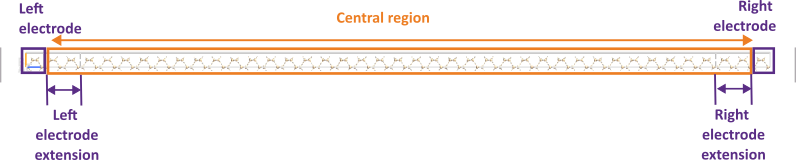### Fitting the TB09-MGGA c-parameter¶

We use the TB09 meta-GGA functional [cTB09] to calculate the projected local density of states and transmission spectrum of the device. In order to obtain an accurate description of the Si side of the interface, the TB09 c-parameter is fitted to match the silicon band gap. Details about this procedure can be found in the tutorial Meta-GGA and 2D confined InAs. The calculations are quite fast and can be run locally.

1. Compute the band structure of the optimized silicon bulk using ATK-DFT with the TB09-MGGA functional and 11x11x11 k-points. You should obtain a band gap of 1.16 eV and a self-consistently determined c-parameter of 1.0841.

2. Do similar calculations with fixed c-values around 1.0841. This can be done in a single QuantumATK Python script by looping over the different c-values. Use the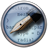Editor to insert the loop:

for tb09 in (1.05,1.055,1.06,1.065,1.07,1.075,1.08,1.085,1.09,1.095,1.1):
# -------------------------------------------------------------
# Calculator
# -------------------------------------------------------------
#----------------------------------------
# Exchange-Correlation
#----------------------------------------
exchange_correlation = MGGA.TB09LDA(c=tb09)


Remember that the Bandstructure block should be included in the loop, just below the Calculator section. Use nlsave('Si_bulk_fitc.nc', bandstructure) to save all the band structures in a single data file.

3. Fit the c-value to the experimental band gap of 1.17 eV [cKit04] using the script c_fit.py. It needs three input parameters; a list of the used c-values (tb09), the name of the .nc file containing the bandstructure calculations (fname), and the experimental band gap (SK).

The optimal c-value should be around 1.0881.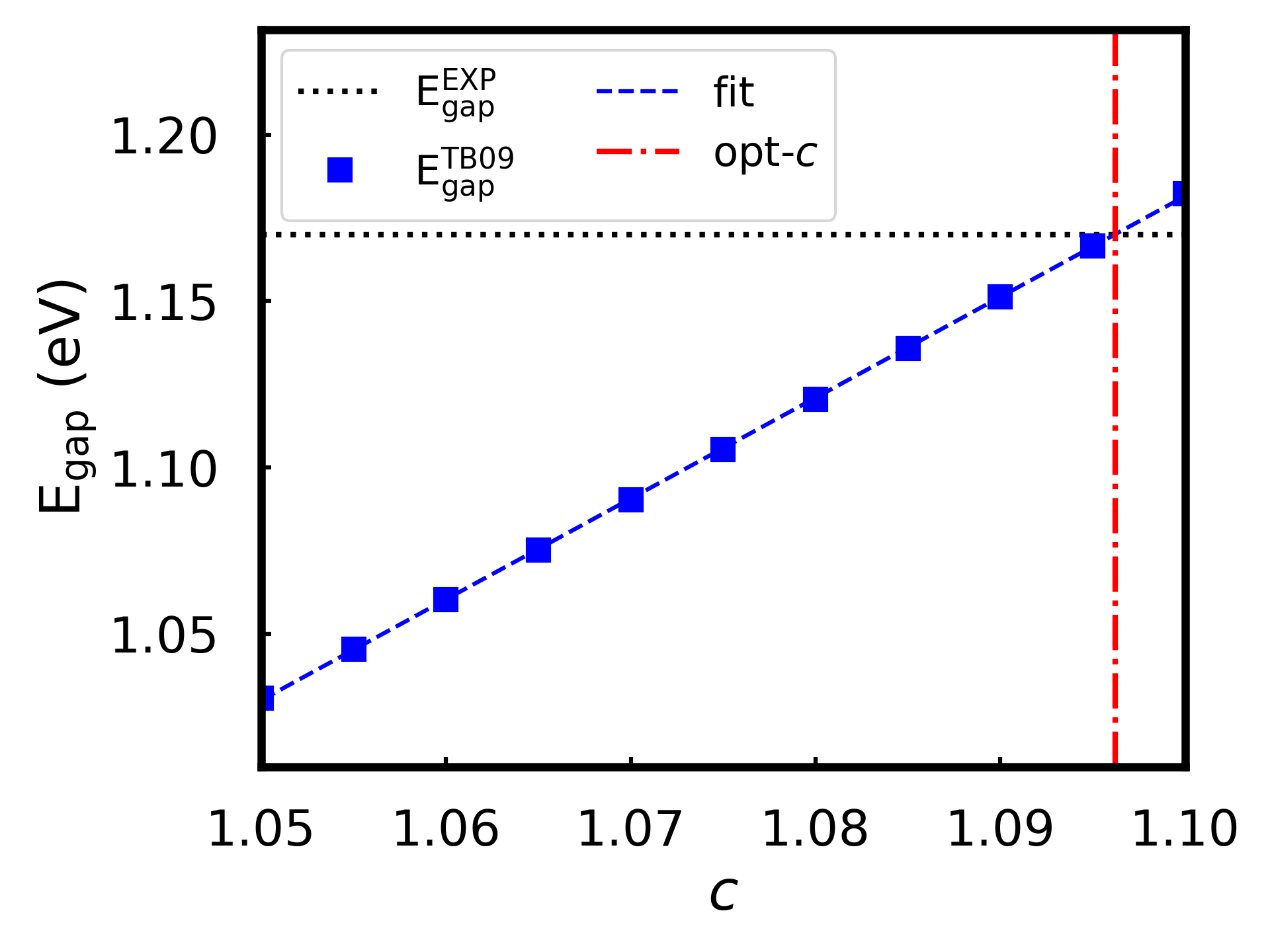### Silicon doping and depletion layer length¶

You are now ready to dope the silicon part of the device. Open the relaxed device configuration in theBuilder, select all the silicon atoms, and use the Doping plugin to apply an n-type doping concentration of 1019 e/cm3 to the right electrode.

It is important to consider the length of the depletion layer in your device: The device needs to be longer than the screening length of the semiconductor so that the potential on the silicon side at the boundary with the silicon electrode resembles that of bulk silicon. This can be done by studying the convergence of the Hartree potential with respect to the length of the silicon central region. For a good starting guess, you can consult some typical depletion layer lengths for metal–semiconductor interfaces [cKP05]; a doping of 1019 cm-3 corresponds to a depletion layer length of around 100 Å.Tip

You only need to converge the length of the silicon side of the interface, since the metal have a much shorter screening length. The 6 atomic layers of Ag will be sufficient.

Note

In QuantumATK versions 2015 and older, theHartreeDifferencePotential analysis object was called ElectrostaticDifferencePotential.

Increase the length of the silicon part of the central region by using the Central Region Size plugin. Use lengths of 80 Å, 100 Å, 120 Å, and 140 Å, and send each device configuration to theScripter. Use a calculator with the same parameters as for the device relaxation, and add theHartreeDifferencePotential block.

Note that the calculations become increasingly difficult to converge as the length is increased. At 120 Å and more, you will need to adjust the Iteration Control Settings in your calculator: In the Scripter window, change the Script detail to Show defaults, and send the script to theEditor. In the Iteration Control Settings block, change the values of damping_factor, number_of_history_steps, and max_steps used in the device calculation:

device_iteration_control_parameters = IterationControlParameters(
damping_factor=0.05,
linear_dependence_threshold=0.0,
algorithm=PulayMixer(),
preconditioner=Preconditioner.Off,
start_mixing_after_step=0,
number_of_history_steps=12,
max_steps=400,
tolerance=0.0001,
mixing_variable=HamiltonianVariable,
)


The calculation for the largest device requires around 13 hours on 16 cores. When all calculations have finished and the results appear on the LabFloor, you can plot the potentials along the Z-direction using the 1D projector analysis tool. The potential should be flat in the vicinity of the boundary with the silicon electrode.The wiggles of the potential can make it hard to see if it has converged to the electrode value. The macroscopic average of the potential, $$\langle \Delta V_H \rangle$$, smoothens out the wiggles. Use the script hdp.py to calculate the macroscopic average of the Hartree potential along the z-direction [cBBR88]. The script needs to be edited, as it contains some input parameters specific to the interface structure:

gauss_left: Distance along Z (in Å) between two consecutive atomic layers in the left electrode material. Same as above, but for the right electrode material. Z-coordinate of the interface position (in Å) calculated as the midpoint between the last atom in the left-hand material and the first atom in the right-hand material. Name for the output .dat file containing the averaged potential.

Once you have edited these parameters, run the script once for each of the the .nc files containing the HartreeDifferencePotential analysis for the different configurations:

atkpython hdp.py device.nc


where device.nc should be changed to the actual .nc file name.

You can use the script hdp_plot.py to compare the resulting .dat output files. Modify the following input parameters:

fnames: Names of the .dat files. Lenghts of the silicon central region. Same as explained above (input for hdp.py).

Edit the script and run it to produce the figure below:

atkpython hdp_plot.py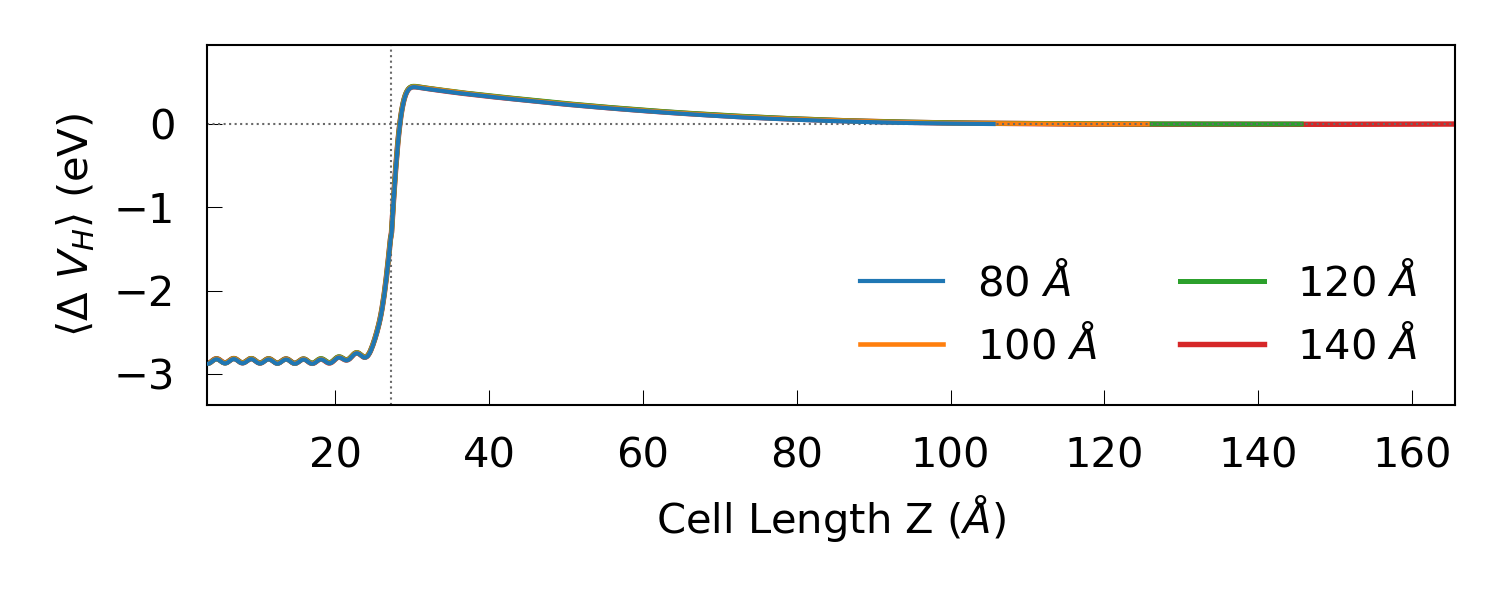We may conclude from the plot that a silicon central region length of 120 Å should be sufficient. Note that the depletion layer length could change slightly with the bias. You now have the final device.py.

Note

The kink at the interface border in the plot above is due to the averaging procedure, where Gaussians of different widths are matched at the Ag–Si border.

## Projected local density of states¶

The projected LDOS (PLDOS) offers a highly useful visualization of the band diagram of the interface. Use the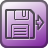Analysis from File block in theScripter to load the saved device configuration and the converged calculator attached to it. Then add theProjectedLocalDensityOfStates block and edit the settings: Energy range between -2 and +2 eV using 401 energy points and a 18x9 k-point grid. Save the results in the .nc file from which you load the configuration.

The calculation will take around 7 hours on a 16-core node with 64 GB of memory. When the calculation is finished and the results are on the LabFloor, you can use the Projected Local Density of States analysis plugin to plot the PLDOS along the device transport direction: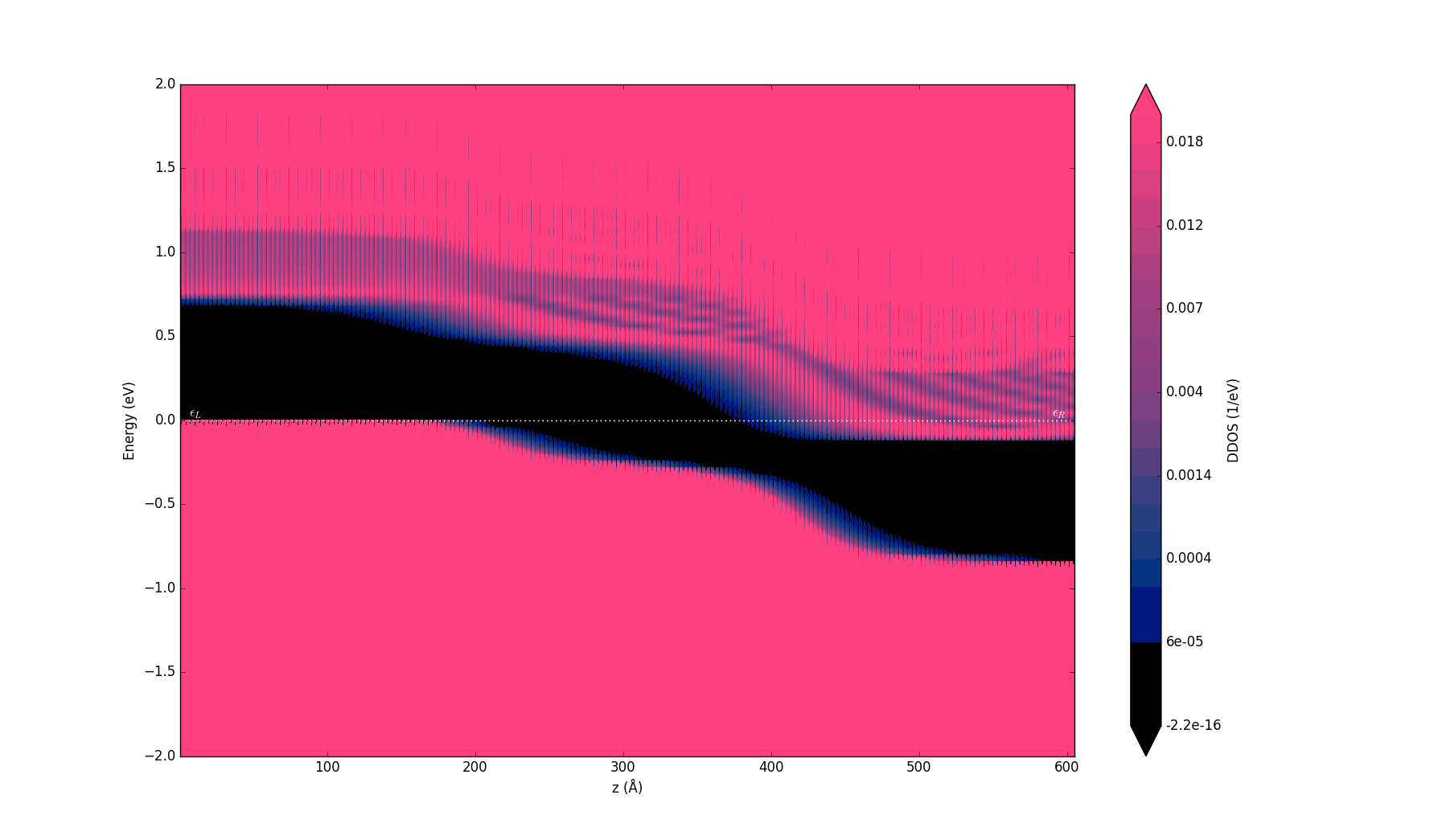You can also plot the averaged Hartree difference potential along with the PLDOS using the script pldos_hdp.py. It requires the .nc file containing both the HartreeDifferencePotential and ProjectedLocalDensityOfStates analysis items, and the same input parameters as hdp.py, along with some plotting preferences:

leftname: Name of the left-hand interface material. Name of the right-hand interface material. The amount of left material shown in the plot (in Å).

Run the script as atkpython pldos_hdp.py device.nc using the generated .nc file.In this plot, you can easily see the metal-induced gap states to the right of the interface border. Moreover, the averaged potential nicely follows the conduction band minimum, both at the interface and far from it. We can therefore estimate the Schottky barrier, $$\Phi^{pot}$$, by calculating the difference between the chemical potential of the left electrode, $$\mu_L$$, and the maximum of $$\langle \Delta V_H \rangle$$. The plotting script returns this value together with the plot. You will get a barrier of around 0.606 eV, which is in agreement with experimental results, see [cBML13] and [cGASL10]. The script also returns the position of the conduction band minimum with respect to $$\mu_L$$. You will need this value later on for analyzing the spectral current.

## Finite-bias calculations¶

We will investigate the I–V characteristics next. Use theAnalysis from File functionality and theIVCurve analysis block. Choose the following settings for the analysis:

• -0.3 to +0.3 V bias range with 15 points.
• -2 to +2 eV energy range with 401 points.
• 18x9 k-point grid.

The calculation will require around 15 hours on 16 cores. You can use the IV-Plot plugin to plot the calculated current vs. bias, or use the script IV.py to plot the current density against bias. The script requires a .nc file containing the device configuration and I–V curve items.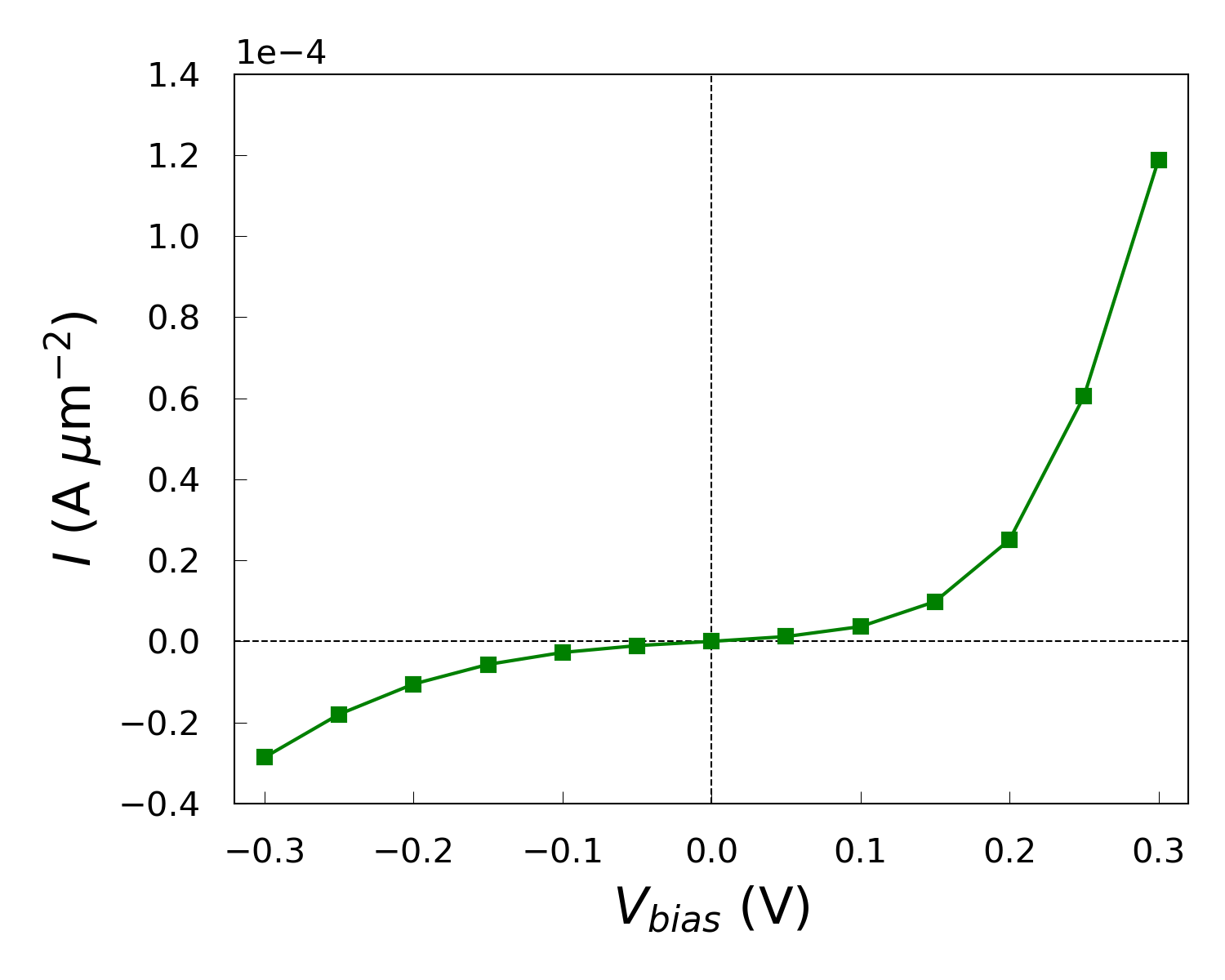The interface shows Schottky diode-like behavior, with a large increase in the current at forward bias but a rather small increase at reverse bias.

Note

The computed current is significantly lower than the current reported in the publication [cSMB+16]. See the Note on the variation of the current for an explanation.

### Ideality factor¶

Thermionic emission theory describes the I–V characteristics of a Schottky diode as [cSK06]

$I = I_0 \mathrm{e}^{\frac{qV_{bias}}{nk_BT}} \left(1-\mathrm{e}^{-\frac{qV_{bias}}{k_BT}}\right),$

where $$q$$ is the elementary charge, $$k_B$$ is the Boltzmann constant, $$T$$ is the temperature, $$I_0$$ is the saturation current, and $$n$$ is the so-called ideality factor. The ideality factor is a measure of how much the interface resembles an ideal Schottky diode, where a value of $$n$$=1 represents the ideal case. Using a log-plot of $$I/(1-\mathrm{e}^{-qV_{bias}/k_BT})$$ against $$V_{bias}$$ you can extract the value of the ideality factor from the slope. Use the script IV-n-log.py to create such a plot and calculate the ideality factor. You should edit a few input parameters:

filename: The same .nc file as for IV.py. The temperature at which the calculations have been run. The number of bias points to include in the fit used to find the ideality factor.

Running the script, you should get a value around $$n$$=1.8208, which means that the system deviates significantly from the ideal Schottky diode behavior.### Schottky barrier¶

The Activation Energy (AE) method [cSK06] is a widely used experimental procedure for extracting the Schottky barrier. The method extracts the Schottky barrier from an Arrhenius-like plot of $$\ln(I/T^2)$$ vs. $$1/T$$,

$\ln(IT^2) = \ln(AA^*) - \frac{q}{k_B} \left( \Phi^{AE} - \frac{V_{bias}}{n} \right) \frac{1}{T} \Rightarrow$
$\phi^{AE}(V_{bias}) = \Phi^{AE} - \frac{V_{bias}}{n} = -\frac{k_B}{q} \frac{\mathrm{d}[\ln(I/ T^2)]}{\mathrm{d}(1/T)}.$

Use the script IvsT.py to calculate the transmission at each applied forward bias in a range of temperatures between 250 K and 400 K. The transmission is calculated in a linear-response fashion using the Landauer–Büttiker expression for the current,

$I = \frac{2q}{h} \int T(E,\mu_L,\mu_R) \left[ f \left( \frac{E-\mu_L}{k_BT} \right) - f \left( \frac{E-\mu_R}{k_BT} \right) \right] \mathrm{d}E,$

where the transmission coefficient $$T(E,\mu_L,\mu_R)$$ has been evaluated self-consistently at a temperature of 300 K during the the IVCurve calculation.

The script needs three inputs to run:

fname: The .nc file containing the IVCurve analysis. Maximum temperature used in the calculations (in K). Minimum temperature used in the calculations (in K).

Running the script produces a .dat file containing the I–T characteristics for each of the forward bias points. From these files, you can create the simulated Arrhenius plot using arrhenius.py. It requires the Tmax and Tmin inputs, and also the following:

IF: The calculated ideality factor from IV-n-log.py. Forward biases for which the calculations have been performed (in V). Name of output file containing the effective barrier height (in eV).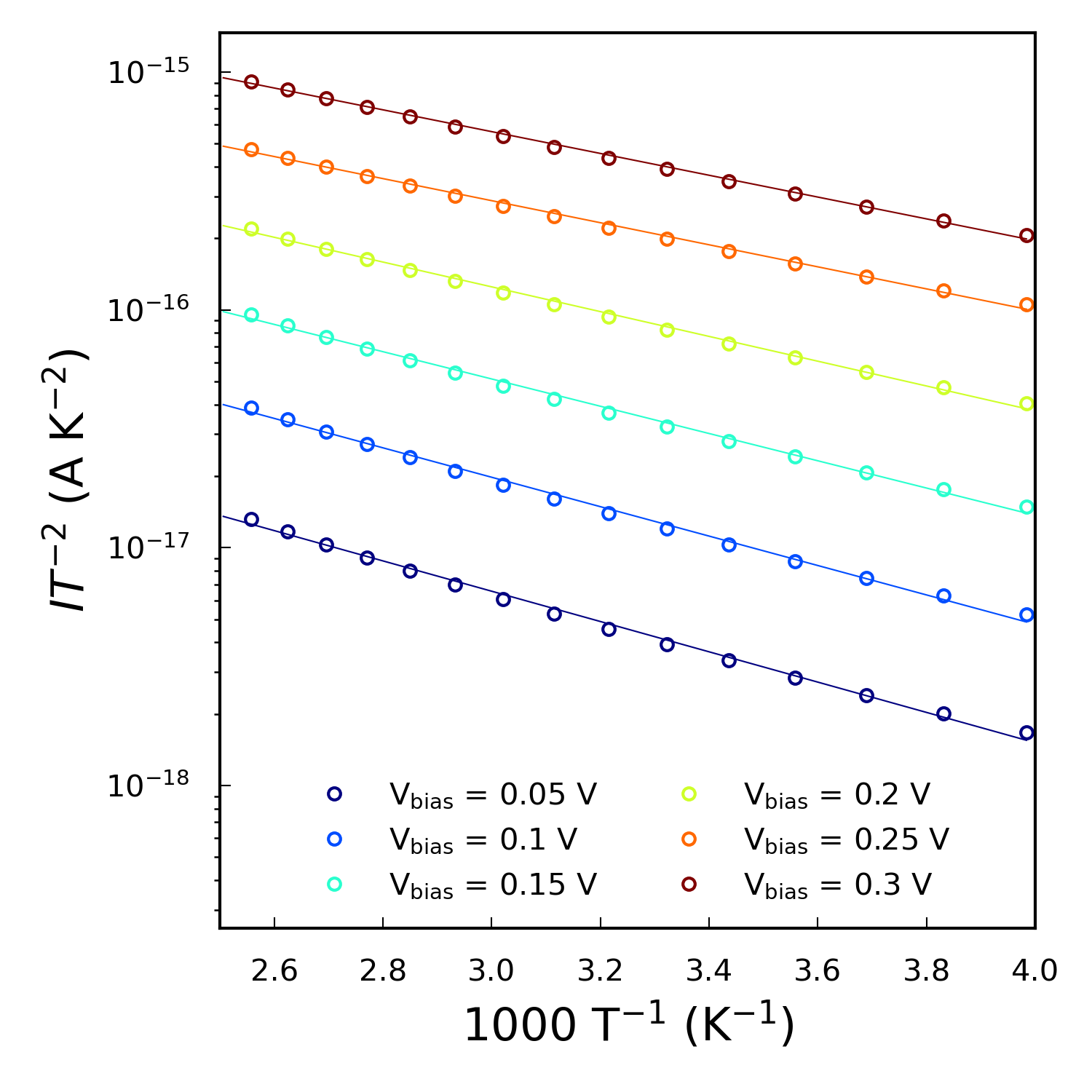The script produces the plot above, and also returns the Schottky barrier height $$\Phi^{AE}$$ and the effective barrier height $$\phi^{AE}(V_{bias})$$ for each applied bias ($$\phi^{AE}$$ is the barrier from the conduction band minimum of the bulk silicon electrode to the maximum of $$\langle V_H\rangle$$). The effective barrier height is exported as a .dat file for later analysis. You will find that the Schottky barrier increases with bias voltage. This is unphysical and should not occur, which suggests that the AE method provides only a limited description of this interface.

### Spectral current¶

The final analysis is an investigation of the spectral current,

$I(E) = \frac{2q}{h} T(E,\mu_L,\mu_R) \left[ f \left( \frac{E-\mu_L}{k_BT} \right) - f \left( \frac{E-\mu_R}{k_BT} \right) \right].$

We will plot the spectral current and the averaged Hartree difference potential together in order to compare the energy of maximum spectral current with the barrier of the Hartree potential.

The starting point is to calculate the Hartree potential at the different biases considered. Do this usingAnalysis from File and load the configurations saved in the file ivcurve_selfconsistent_configurations.nc, which was created by the IVCurve calculation, and add theHartreeDifferencePotential block for each configuration. The calculations should be very fast and can be run locally. You can then reuse hdp.py to calculate the average of each of the potentials.

Use the script spectral_current.py to plot the averaged potential together with the spectral current. You need to edit a few parameters:

fname: The .nc file containing the IVCurve analysis. The .dat files containing the averaged potentials. Schottky barrier calculated with pldos_hdp.py at zero bias (in eV). Conduction band minimum of the bulk Si electrode with respect to $$\mu_L$$, calculated above with pldos_hdp.py (in eV). Name of the output file containing the barriers associated with the thermionic emission process (in eV). Name of the output file containing the energy of maximum spectral current (in eV).

Running the script should produce the figure below.The script also returns the estimated barrier for thermionic emission, $$\phi_F$$, and the energy of maximum spectral current for each bias as .dat files. The energy axis of the two plots is aligned by plotting the averaged potential relative to the electron affinity of bulk Si (estimated by pldos_hdp.py) and $$\mu_L$$ and the spectral current relative to $$\mu_L$$.Transmission through the device can occur through two different processes; either by thermionic emission or by tunneling through the Schottky barrier. If the transmission occurs solely by thermionic emission, the spectral current would be zero below $$\Phi^{pot}$$. This is definitely not the case. In fact, the energy of maximum spectral current is below the thermionic emission barrier for each bias, revealing that the dominant transmission process is tunneling!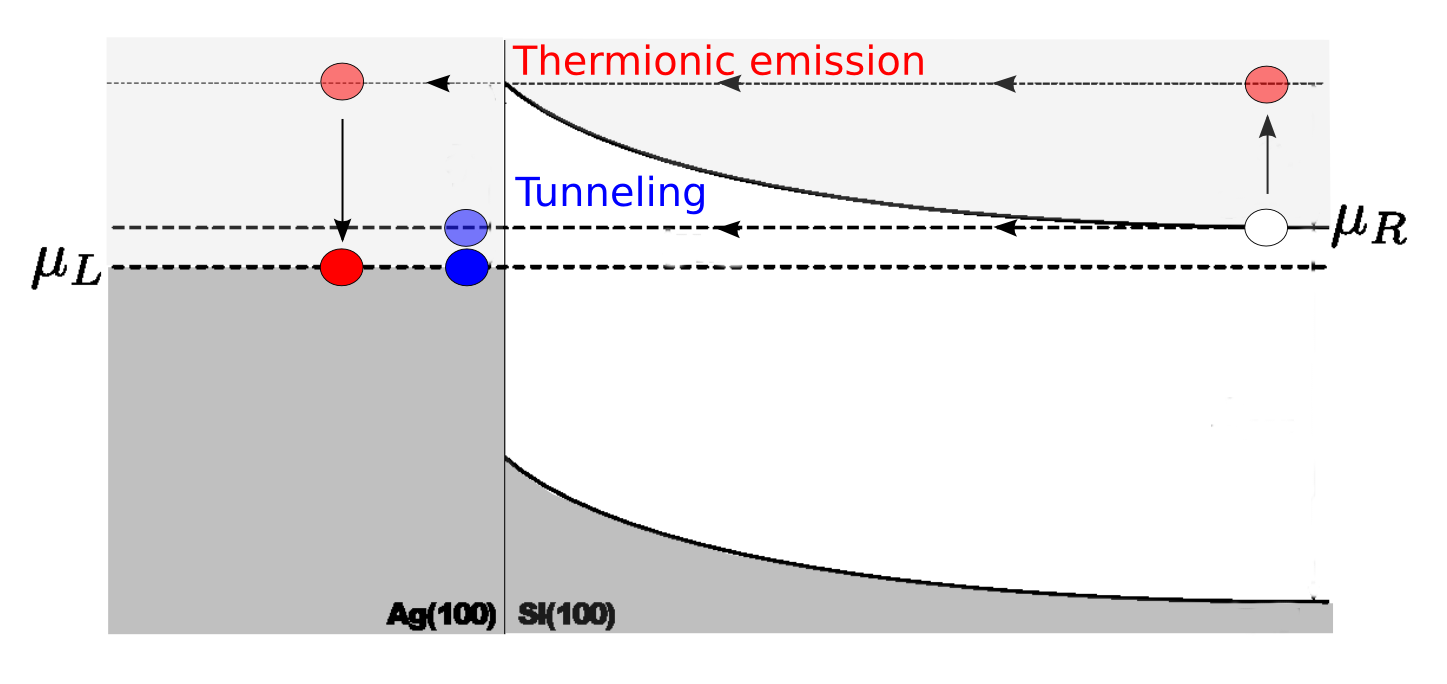### Summing up the results¶

We compare the finite-bias results by plotting the following:

Create the plot using barrier_compare.py. It requires the .dat files created by arrhenius.py and spectral_current.py along with the calculated ideality factor from IV-n-log.py and the estimated Schottky barrier at zero bias, $$\Phi^{pot}$$, from pldos_hdp.py.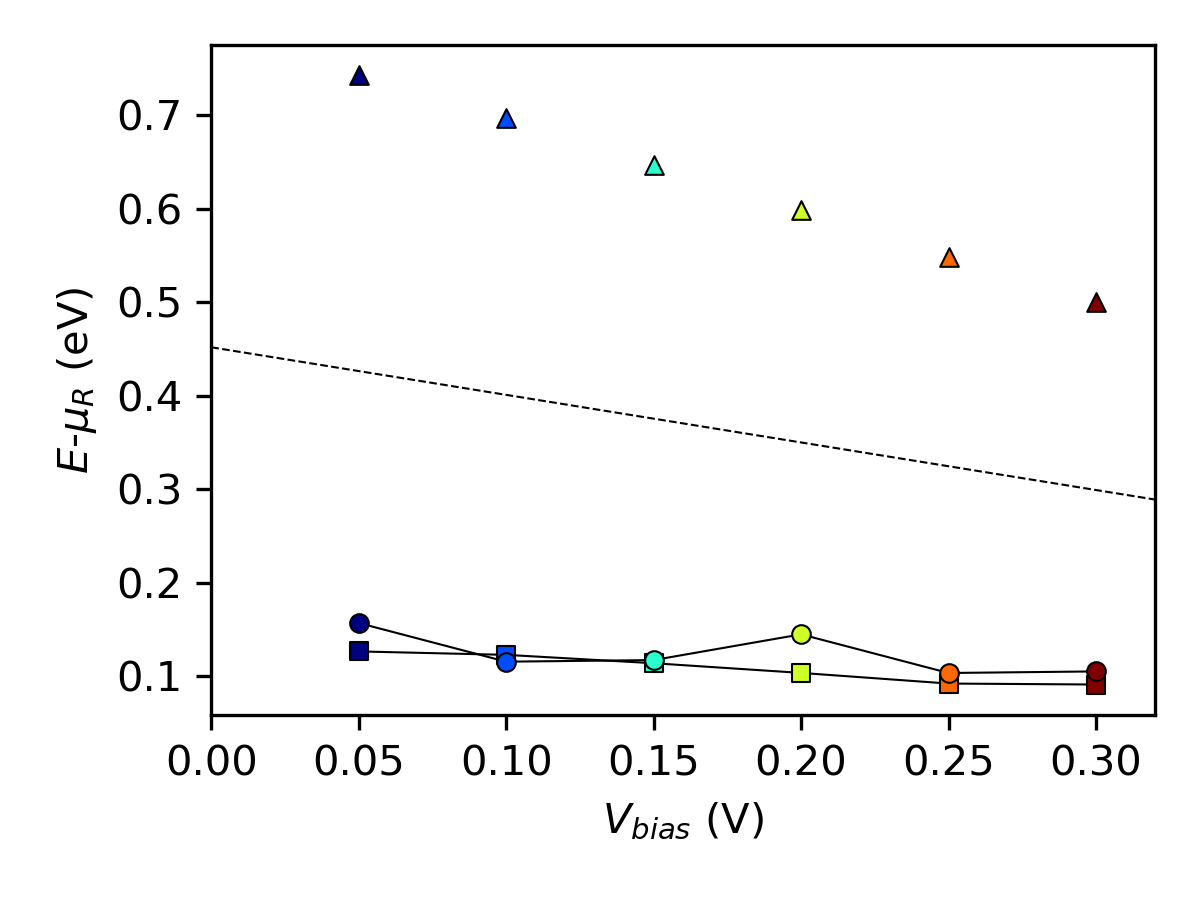The plot shows that the estimated AE Schottky barrier (squares) lies below the thermionic emission barrier (triangles) for each bias point. This is because the AE method neglects contributions from tunneling and thereby implicitly assumes that the maximum spectral current (circles) will occur at the energy of the barrier height. Furthermore, we see that the analytical bias dependence of the thermionic emission barrier (dashed line) is in good agreement with the calculated barrier heights (triangles).

## Note on the variation of the current¶

The calculated current is two orders of magnitude lower than the current reported in the publication [cSMB+16]. This is due to the use of two slightly different Ag electrodes: The silver electrode used in the article was not relaxed after matching the two surfaces at the interface – that electrode is therefore compressed as compared to the Ag electrode used in this case study. This has an impact on the electronic structure of the electrode, which can be shown by comparing the electrode bandstructures:In the figure above, “electrode_ref.nc” is the electrode used in the article, while “electrode_used.nc” denotes the electrode used here, and “electrode_bulk.nc” is a completely unstrained silver electrode. Comparing the first two, we see that the elongation of the electrode generally results in lower energy levels. The electrode used in this case study has fewer k-points (and thereby states) available in the bias window, which results in smaller transmission and thereby a lower current. However, if we compare the first two bandstructures to that of unstrained silver, we see that the main features around the Fermi level are preserved with both the electrode used here and the one used in the publication.